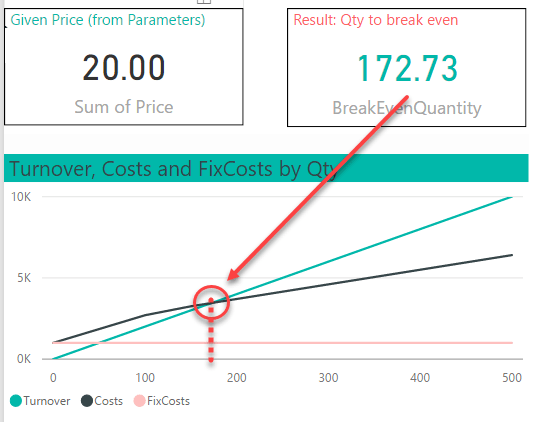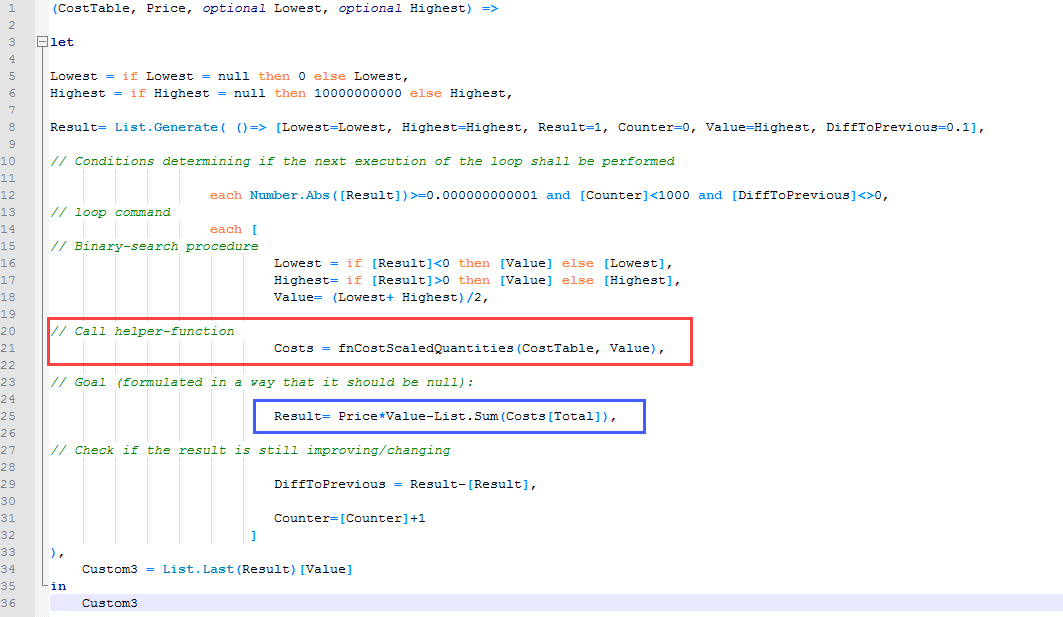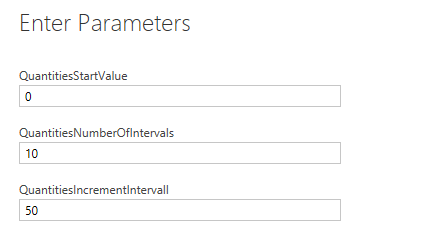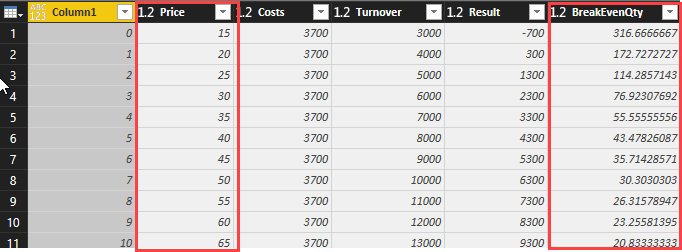# Non-linear Break-Even Analysis in PowerBI

A break-even analysis tells you at which value of the parameter in question your profit-calculation will turn positive (link). Here we need to sell at least 173 at a given price of 20 before we’ve recovered all our costs:If your variable costs are constant, you can solve it by this formula:

`BreakEvenQuantity = Total Fixed Costs / (Unit Sale Price - Unit Variable Costs)`

You’ll find tons of examples on how to do this in Excel .

### Non-linear cost structure

But in real life, the variable costs often depend on certain quantities as you get discounts for purchasing large amounts. The following table shows a cost structure with fix costs in row 1. The 3rd column “FixOrQty” indicates if the cost item is fix or dependent on the quantity (Qty). The 2nd row contains a variable cost that is constant with 2 for all quantities. Row 3&4 show a variable cost of 8 for quantities up to 100 and if you purchase more than 100 the costs will be lowered to 2 for all additional quantities. Row 5-7 have a similar structure, but with 3 quantity ranges:

### Solve with goal-seek algorithm

If we aim to sell the product for 20 (“Price”), we want to know how much we need to sell at least to recover all our costs (“Value”). To solve this, we adjust the goal-seeking-function from my XIRR-post as follows:Blue: The goal that should be 0 is the “Result”. “Value” is the target value quantity, so the first argument: “Price*Value” returns the turnover. “List.Sum(Costs[Total])” is our costs as the sum of the column “Total” from the variable “Costs”. That comes from our helper-function marked in red:

### Allocating scaled quantities

This function splits up the current quantity (200 in the example below) onto the specific discount ranges in column “RelevantQty”. The last column “Total” multiplies the “Amount” with the “RelevantQty” for all variable costs and returns just the “Amount” for Fix costs (“Fix” in column “FixOrQty”):

### Create table from 3 parameters

To create the line chart from above, you have to calculate the results for some quantities between 0 and 500. You can create such a table with a table-creation formula. Just fill in the following parameters:and this formula:will create this table with steps from 0 to 500:will return the required table:

### Check sensitivity with different price-scenarios

But you might not be happy with the 173 minimum-quantity as you don’t expect to sell that much. Then another chart might be useful that shows the break-even-quantities if you adjust your prices:For this chart you create a table with a range of prices and their resulting break-even-quantities with the same logic as before:### Change the variables: Break-Even Analysis on price

You can adopt the method to search for the critical value of other parameters instead. Like searching the break-even-price for a given quantity.

### And for linear models?

Of course, this method will also work for simple linear models, just fill your data into the cost table and leave the last columns blank. And if your data changes or you get more data that has to be considered as well: Just adjust/fill in the CostTable, and if needed, adjust the parameters.

M-Code for all:

Enjoy and stay queryious 😉

### Comments (4) Write a comment

1.VERY good article – thanks !

•Thanks Tristan!
This is where the fun begins 😉

2.Hi Imke,
Wonderful article! It reminded me of my Algebra classes:)
I think the non-linear problem you describe is a bit misleading. It is actually a Linear Programming type of problem and there are more deterministic algorithms that solve it, ie Simplex (https://en.wikipedia.org/wiki/Simplex_algorithm).
As for the goal-seeking approach, is totally relevant for the non-linear problems (https://en.wikipedia.org/wiki/Nonlinear_programming).

I hope that once the MS team releases the What-IF feature to Power BI, we’ll know what’s their take on solving optimization problems in PBI.

Igor

•